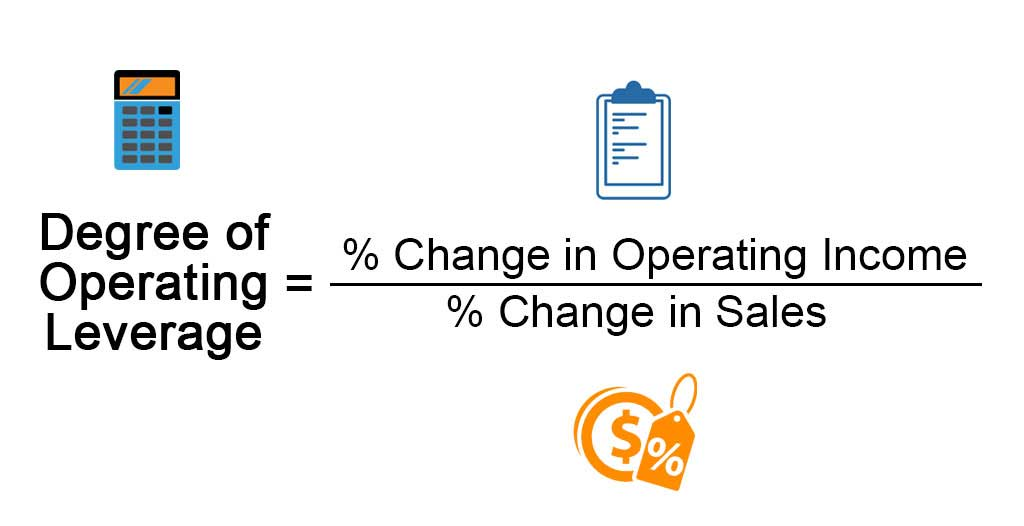## What is Degree of Operating Leverage?

The term “degree of operating leverage” refers to the financial ratio that measures the impact of change in sales on the operating income (EBIT). In other words, this metric helps in assessing the sensitivity of the operating income to the changes in revenue. Basically, the underlying theory for the degree of operating leverage revolves around the mix of variable costs and fixed costs in the overall cost structure of the company.

Theoretically, a high degree of operating leverage for a company indicates that its overall cost structure has a large proportion of fixed costs and as such, any change in sales will result in strong variation in operating profitability. On the other hand, a lower degree of operating leverage means that the company’s profitability is less sensitive to change in sales due to a higher proportion of variable costs in the overall cost structure.

Formula

The formula for the degree of operating leverage can be derived by dividing the percentage change in operating profit by the percentage change in sales. Mathematically, it is represented as,

Degree of Operating Leverage = % Change in Operating Income / % Change in Sales

### Examples of Degree of Operating Leverage (With Excel Template)

Let’s take an example to understand the calculation in a better manner.

You can download this Degree of Operating Leverage Excel Template here – Degree of Operating Leverage Excel Template

#### Example #1

Let us take the example of a company that has achieved total sales of \$80 million during the year 2017, while in the year 2018 the company higher revenue of \$100 million due to a stronger demand for its products. However, the change in the operating expenses was not in line with the revenue growth as the company incurred an operating cost of \$45 million in 2017 and \$55 million in 2018. Determine the degree of operating leverage of the company based on the given information.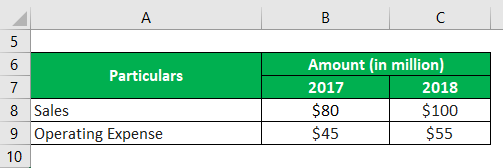Solution:

For 2017

Operating Income is calculated using the formula given below

Operating Income 2017 = Sales 2017 – Operating Expense 2017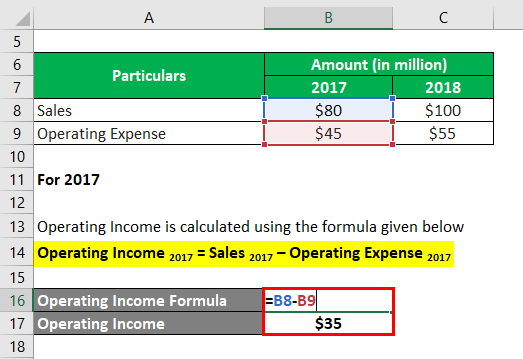•  Operating Income = \$80 million – \$45 million
•  Operating Income = \$35 million

For 2018

Operating Income is calculated using the formula given below

Operating Income 2018 = Sales 2018 – Operating Expense 2018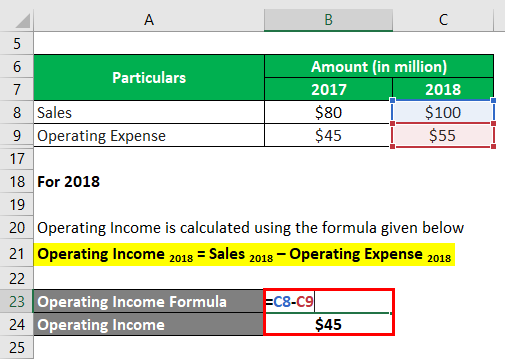• Operating Income = \$100 million – \$55 million
• Operating Income = \$45 million

% Change in Operating Income is calculated using the formula given below

% Change in Operating Income= (Operating Income 2018 – Operating Income 2017) / Operating Income 2017 * 100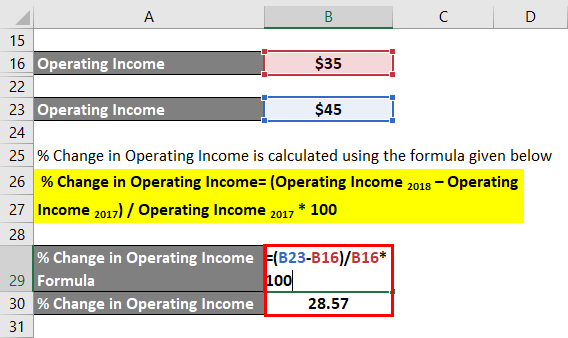•  % Change in Operating Income = (\$45 million – \$35 million) / \$35 million * 100%
•  % Change in Operating Income = 28.57%

% Change in Sales is calculated using the formula given below

% Change in Sales = (Sales 2018 – Sales 2017) / Sales 2017 * 100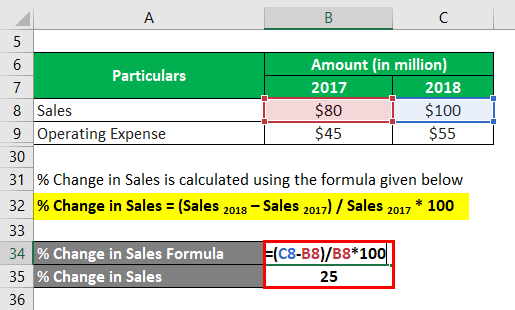•  % Change in Sales = (\$100 million – \$80 million) / \$80 million * 100
•  % Change in Sales = 25%

Degree of Operating Leverage is calculated using the formula given below

Degree of Operating Leverage = % Change in Operating Income / % Change in Sales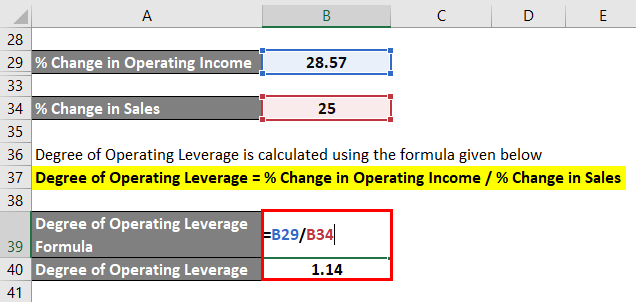• Degree of Operating Leverage = 28.57% / 25.00% = 1.14

Therefore, based on the given information it can be seen that the company’s degree of operating leverage is 1.14.

#### Example #2

Let us take the example of two real-life companies – Samsung and Apple, to compute and check which of these two cell phone giants have higher operating leverage. According to the annual reports of both the companies the following information is available: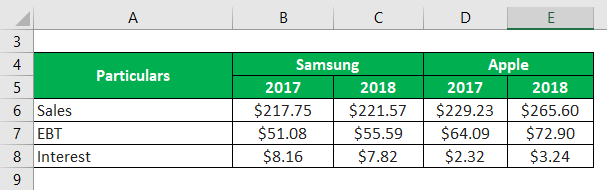Solution:

Operating Income for 2017 is calculated using the formula given below

Operating Income 2017 = EBT 2017 + Interest 2017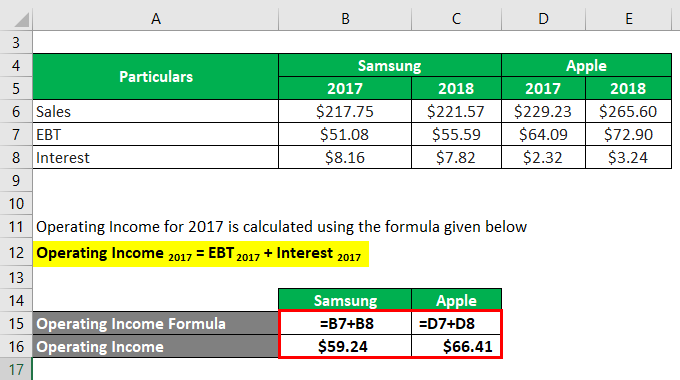Samsung

• Operating Income = \$51.08 billion + \$8.16 billion
• Operating Income = \$59.24 billion

Apple

• Operating Income = \$64.09 billion + \$2.32 billion
• Operating Income = \$66.41 billion

Operating Income  for 2018 is calculated using the formula given below

Operating Income 2018 = EBT 2018 + Interest 2018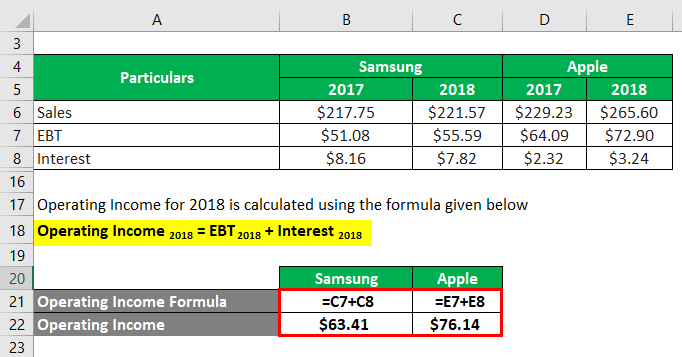Samsung

• Operating Income = \$55.59 billion + \$7.82 billion
• Operating Income = \$63.41 billion

Apple

• Operating Income = \$72.90 billion + \$3.24 billion
• Operating Income = \$76.14 billion

% Change in Operating Income is calculated using the formula given below

% Change in Operating Income = (Operating Income 2018 – Operating Income 2017) / Operating Income 2017 * 100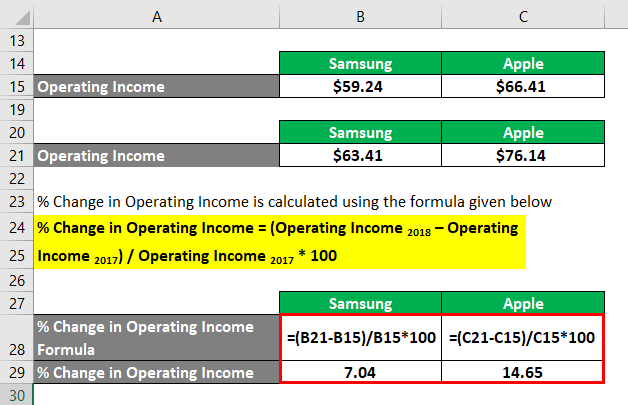Samsung

• % Change in Operating Income = (\$63.41 billion – \$59.24 billion) / \$59.24 billion * 100%
• % Change in Operating Income = 7.04%

Apple

• % Change in Operating Income = (\$76.14 billion – \$66.41 billion) / \$66.41 billion * 100%
• % Change in Operating Income = 14.65%

% Change in Sales is calculated using the formula given below

% Change in Sales = (Sales 2018 – Sales 2017) / Sales 2017 * 100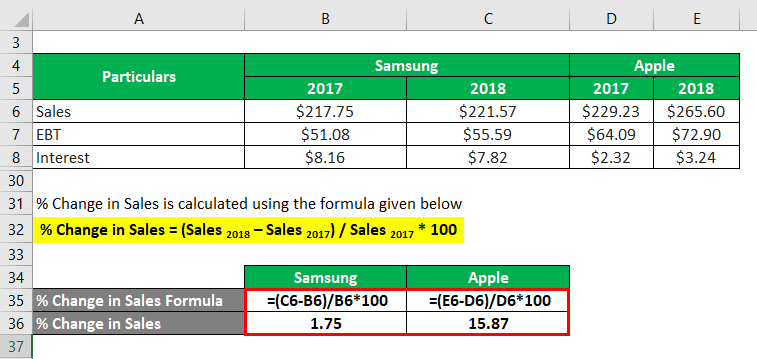Samsung

•  % Change in Sales = (\$221.57 billion – \$217.75 billion) / \$217.75 billion * 100%
•  % Change in Sales = 1.75%

Apple

• % Change in Sales = (\$265.60 billion – \$229.23 billion) / \$229.23 billion * 100%
•  % Change in Sales = 15.87%

Degree of Operating Leverage is calculated using the formula given below

Degree of Operating Leverage = % Change in Operating Income / % Change in Sales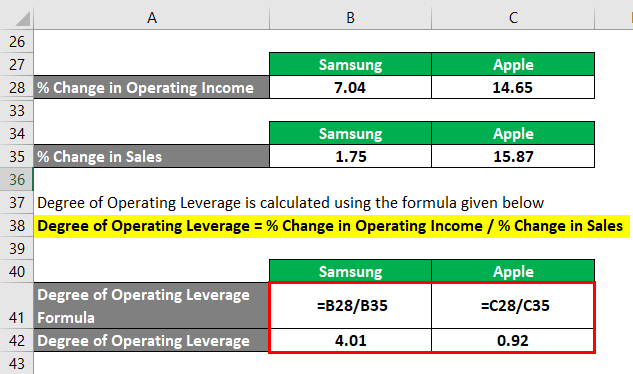Samsung

• Degree of Operating Leverage = 7.04% / 1.75% = 4.01

Apple

• Degree of Operating Leverage = 14.65% / 15.87% = 0.92

Therefore, Samsung’s % of operating leverage stood at 4.01 compared to 0.92 of Apple which means a larger proportion of fixed costs in its cost structure.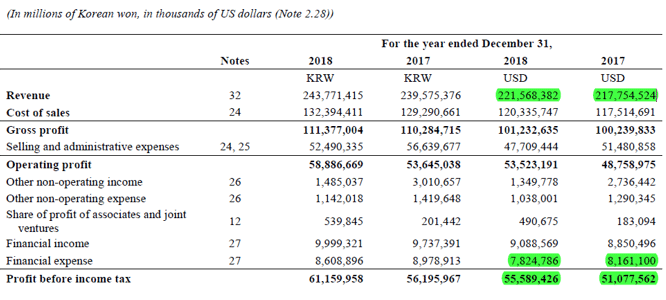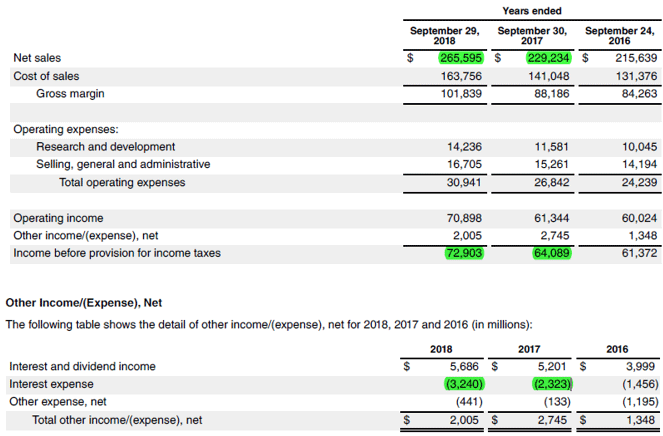Source Link: Apple Inc. Balance Sheet

### Conclusion

So, it can be concluded that the degree of operating leverage helps in assessing how well a company can leverage its cost structure to drive its operating profitability. Please note that a high degree of operating leverage is a huge positive when the sales are growing, while it can be detrimental to the profitability of a company with declining revenue.

### Recommended Articles

This is a guide to the Degree of Operating Leverage. Here we discuss how to calculateDegree of Operating Leverage along with practical examples. We also provide a downloadable excel template. You may also look at the following articles to learn more –

The post Degree of Operating Leverage appeared first on EDUCBA.